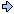World!Of
NumbersWON plate
22 |

[ July 16, 2001 ]
18812 = 3538161
This square falls apart into two primes namely 353 and 8161.
Note that 8161 is the smallest prime one has to append to palprime 353
so that their concatenation yields a square !1881 is the middle term of the smallest triplet of consecutive HAPPY numbers.
What is a happy number ?
Source : David Wells, "Curious and Interesting Numbers", revised edition, 1997, p. 154.
[ 1880, 1881, 1882 ]
1881 and Sum Of Square Of Digits = 130
130 and Sum Of Square Of Digits = 10
10 and Sum Of Square Of Digits =   1if One then Happy !John Venn published Symbolic Logic in 1881.
Source
This historical fact was brought to my attention by G. L. Honaker, Jr..The palindrome 1881 can be expressed
by using the six consecutive integers 2, 3, 4, 5, 6 and 7
in the following construction :

1881 = 27 + 36 + 45

The exponents 7, 6, 5 are the continuation of the basenumbers 2, 3, 4 in reversed order
Note that 234 + 765 = 999 another palindrome !

WARNING  you will have a hard time finding another palindrome with such properties.21881 + 1881 is probable prime ! (Sloane's A052007)1881 features in this remarkable Magic Square
from Ivars Peterson's MathTrek 'Math Trails in Ottawa'

8888111811818811
1811818181181888
8111188118188188
1188881888811111

Hold this magic square in a mirror
and you'll see it keeps its magic property!The Number_Of_Digits of {666^666} = 1881
The Number_Of_Digits of {66^66} = 121
Note that 1881 + 121 = 2002
the last palindromic year of this century... of our life ?1881 is a Generalized Catalan Number !
Sloane's A023428 tells us so!There are 1881 partitions of 37 into prime parts (1 included).
(from Sloane's A034891)
Who can give some illustrative examples ?

Coincidentally 1881 is pseudoprime to base 37 ! (A020165)1881 is the sum of 4 distinct positive cubes in exactly 2 ways. (A025409)
Solution : 13 + 33 + 53 + 123 = 1881 = 33 + 53 + 93 + 103

Coincidentally 1881 has exactly 4 prime factors, 2 of which are the same !
1881 = 3 x 3 x 11 x 19

Coincidentally the digit sums on both sides of the equation are equal !Mustafa Kemal (aka Atatürk), founder of the Turkish Republic, was born in 1881,
in Salonica, then an Ottoman city, now in Greece.
( ps. he died in 1938 in room number 71 )The largest (probable) prime of  2184 digits is made with 1881
as the negative displacement from that power of ten axis

Note that the difference between the exponent and the
displacement is also palindromic (21841881 = 303)

The smallest (probable) primes made with 1881
as the positive displacement from its power of ten axis are :

 102184 – 1881 108047 + 1881 1010417 + 1881 1019503 + 1881A000022Prime Curios!Prime PuzzleRichard PhillipsWikipedia 22Le Nombre 22Numberland 22```

```

[TOP OF PAGE]

Patrick De Geest - Belgium- Short Bio - Some Pictures